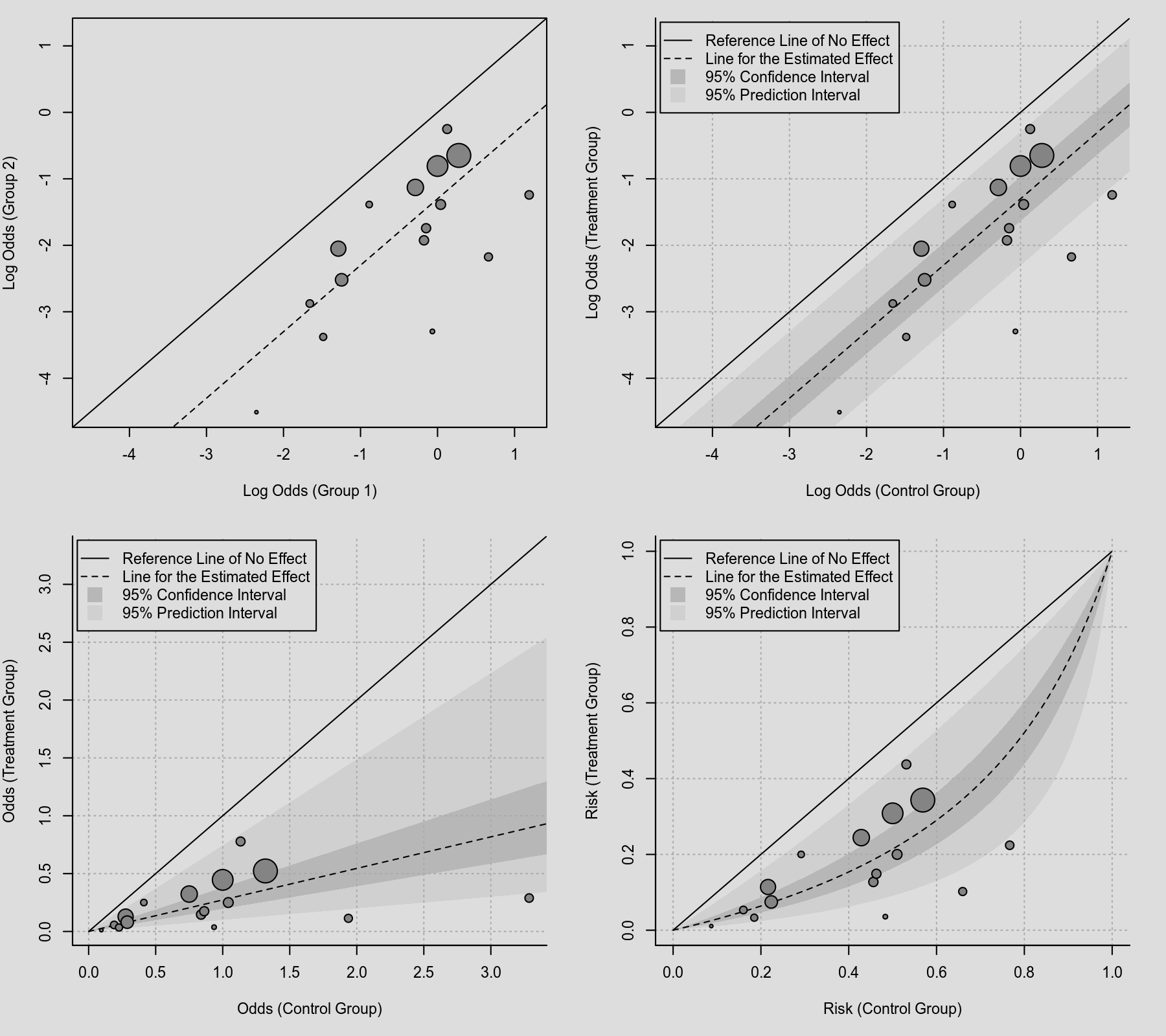#The metafor Package

A Meta-Analysis Package for R

plots:labbe_plot

## L'Abbé Plot

### Description

In a L'Abbé plot (based on L'Abbé, Detsky, & O'Rourke, 1987), the arm-level outcomes for two experimental groups (e.g., treatment and control group) are plotted against each other. In the example below, the points show the log risk (of a tuberculosis infection) in the treatment (x axis) and control (y axis) group. Points falling on the solid diagonal line represent studies where the risk of infection did not differ between the two groups. Points falling below this line represent studies where the risk was lower in the treated (vaccinated) group. The dashed line indicates the estimated effect based on the fitted model (which is linear on the log scale for the log risk ratio). The size of the points is an inverse function of the precision of the estimates (so larger points correspond to more precise estimates).

### Plot### Code

library(metafor)

### decrease margins so the full space is used
par(mar=c(5,4,1,2))

### fit random-effects model
res <- rma(ai=tpos, bi=tneg, ci=cpos, di=cneg, data=dat.bcg, measure="RR")

### draw L'Abbé plot
labbe(res)

L'Abbé, K. A., Detsky, A. S., & O'Rourke, K. (1987). Meta-analysis in clinical research. Annals of Internal Medicine, 107(2), 224–233.

### Page Tools